# mne.Covariance#

class mne.Covariance(data, names, bads, projs, nfree, eig=None, eigvec=None, method=None, loglik=None, *, verbose=None)[source]#

Noise covariance matrix.

Note

This class should not be instantiated directly via `mne.Covariance(...)`. Instead, use one of the functions listed in the See Also section below.

Parameters:
dataarray_like

The data.

names

Channel names.

projs`list`

Projection vectors.

nfree`int`

Degrees of freedom.

eig

Eigenvalues.

eigvec

Eigenvectors.

method

The method used to compute the covariance.

loglik`float`

The log likelihood.

verbose

Control verbosity of the logging output. If `None`, use the default verbosity level. See the logging documentation and `mne.verbose()` for details. Should only be passed as a keyword argument.

Attributes:
`data``array` of shape (n_channels, n_channels)

Numpy array of Noise covariance matrix.

`ch_names`

Channel names.

`nfree``int`

Number of degrees of freedom.

dim`int`

The number of channels `n_channels`.

Methods

 `__add__`(cov) Add Covariance taking into account number of degrees of freedom. `__contains__`(key, /) True if the dictionary has the specified key, else False. `__getitem__` x.__getitem__(y) <==> x[y] Implement iter(self). Return len(self). Set covariance to be processed as being diagonal. Copy the Covariance object. `fromkeys`(iterable[, value]) Create a new dictionary with keys from iterable and values set to value. `get`(key[, default]) Return the value for key if key is in the dictionary, else default. `pick_channels`(ch_names[, ordered, verbose]) Pick channels from this covariance matrix. `plot`(info[, exclude, colorbar, proj, ...]) Plot Covariance data. `plot_topomap`(info[, ch_type, scalings, ...]) Plot a topomap of the covariance diagonal. `pop`(key[, default]) If the key is not found, return the default if given; otherwise, raise a KeyError. Remove and return a (key, value) pair as a 2-tuple. `save`(fname, *[, overwrite, verbose]) Save covariance matrix in a FIF file. `setdefault`(key[, default]) Insert key with a value of default if key is not in the dictionary. `update`([E, ]**F) If E is present and has a .keys() method, then does: for k in E: D[k] = E[k] If E is present and lacks a .keys() method, then does: for k, v in E: D[k] = v In either case, this is followed by: for k in F: D[k] = F[k]

Add Covariance taking into account number of degrees of freedom.

__contains__(key, /)#

True if the dictionary has the specified key, else False.

__getitem__()#

x.__getitem__(y) <==> x[y]

__iter__(/)#

Implement iter(self).

__len__(/)#

Return len(self).

as_diag()[source]#

Set covariance to be processed as being diagonal.

Returns:
cov`dict`

The covariance.

Notes

This function allows creation of inverse operators equivalent to using the old “–diagnoise” mne option.

This function operates in place.

property ch_names#

Channel names.

clear() None.  Remove all items from D.#
copy()[source]#

Copy the Covariance object.

Returns:
covinstance of `Covariance`

The copied object.

Examples using `copy`: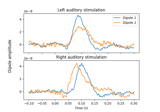Computing source timecourses with an XFit-like multi-dipole model

Computing source timecourses with an XFit-like multi-dipole model
property data#

Numpy array of Noise covariance matrix.

fromkeys(iterable, value=None, /)#

Create a new dictionary with keys from iterable and values set to value.

get(key, default=None, /)#

Return the value for key if key is in the dictionary, else default.

items() a set-like object providing a view on D's items#
keys() a set-like object providing a view on D's keys#
property nfree#

Number of degrees of freedom.

pick_channels(ch_names, ordered=None, *, verbose=None)[source]#

Pick channels from this covariance matrix.

Parameters:
ch_names

List of channels to keep. All other channels are dropped.

orderedbool

If True (default False), ensure that the order of the channels in the modified instance matches the order of `ch_names`.

New in v0.20.0.

Changed in version 1.5: The default changed from False in 1.4 to True in 1.5.

verbose

Control verbosity of the logging output. If `None`, use the default verbosity level. See the logging documentation and `mne.verbose()` for details. Should only be passed as a keyword argument.

Returns:
covinstance of Covariance.

The modified covariance matrix.

Notes

Operates in-place.

New in v0.20.0.

plot(info, exclude=[], colorbar=True, proj=False, show_svd=True, show=True, verbose=None)[source]#

Plot Covariance data.

Parameters:
info`mne.Info`

The `mne.Info` object with information about the sensors and methods of measurement.

exclude

List of channels to exclude. If empty do not exclude any channel. If ‘bads’, exclude info[‘bads’].

colorbarbool

Show colorbar or not.

projbool

Apply projections or not.

show_svdbool

Plot also singular values of the noise covariance for each sensor type. We show square roots ie. standard deviations.

showbool

Show figure if True.

verbose

Control verbosity of the logging output. If `None`, use the default verbosity level. See the logging documentation and `mne.verbose()` for details. Should only be passed as a keyword argument.

Returns:
fig_covinstance of `matplotlib.figure.Figure`

The covariance plot.

fig_svdinstance of `matplotlib.figure.Figure` | `None`

The SVD spectra plot of the covariance.

Notes

For each channel type, the rank is estimated using `mne.compute_rank()`.

Changed in version 0.19: Approximate ranks for each channel type are shown with red dashed lines.

Examples using `plot`: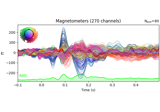Working with CTF data: the Brainstorm auditory dataset

Working with CTF data: the Brainstorm auditory datasetComputing a covariance matrix

Computing a covariance matrix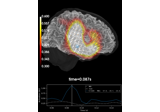Source reconstruction using an LCMV beamformer

Source reconstruction using an LCMV beamformer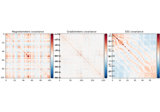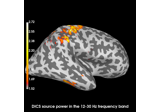Compute evoked ERS source power using DICS, LCMV beamformer, and dSPM

Compute evoked ERS source power using DICS, LCMV beamformer, and dSPM
plot_topomap(info, ch_type=None, *, scalings=None, proj=False, noise_cov=None, sensors=True, show_names=False, mask=None, mask_params=None, contours=6, outlines='head', sphere=None, image_interp='cubic', extrapolate='auto', border='mean', res=64, size=1, cmap=None, vlim=(None, None), cnorm=None, colorbar=True, cbar_fmt='%3.1f', units=None, axes=None, show=True, verbose=None)[source]#

Plot a topomap of the covariance diagonal.

Parameters:
info`mne.Info`

The `mne.Info` object with information about the sensors and methods of measurement.

ch_type‘mag’ | ‘grad’ | ‘planar1’ | ‘planar2’ | ‘eeg’ | `None`

The channel type to plot. For `'grad'`, the gradiometers are collected in pairs and the RMS for each pair is plotted. If `None` the first available channel type from order shown above is used. Defaults to `None`.

New in v0.21.

scalings

The scalings of the channel types to be applied for plotting. If None, defaults to `dict(eeg=1e6, grad=1e13, mag=1e15)`.

projbool | ‘interactive’ | ‘reconstruct’

If true SSP projections are applied before display. If ‘interactive’, a check box for reversible selection of SSP projection vectors will be shown. If ‘reconstruct’, projection vectors will be applied and then M/EEG data will be reconstructed via field mapping to reduce the signal bias caused by projection.

Changed in version 0.21: Support for ‘reconstruct’ was added.

noise_covinstance of `Covariance` | `None`

If not None, whiten the instance with `noise_cov` before plotting.

sensors

Whether to add markers for sensor locations. If `str`, should be a valid matplotlib format string (e.g., `'r+'` for red plusses, see the Notes section of `plot()`). If `True` (the default), black circles will be used.

show_names

If `True`, show channel names next to each sensor marker. If callable, channel names will be formatted using the callable; e.g., to delete the prefix ‘MEG ‘ from all channel names, pass the function `lambda x: x.replace('MEG ', '')`. If `mask` is not `None`, only non-masked sensor names will be shown.

mask`ndarray` of bool, shape (n_channels,) | `None`

Array indicating channel(s) to highlight with a distinct plotting style. Array elements set to `True` will be plotted with the parameters given in `mask_params`. Defaults to `None`, equivalent to an array of all `False` elements.

Additional plotting parameters for plotting significant sensors. Default (None) equals:

```dict(marker='o', markerfacecolor='w', markeredgecolor='k',
linewidth=0, markersize=4)
```
contours

The number of contour lines to draw. If `0`, no contours will be drawn. If a positive integer, that number of contour levels are chosen using the matplotlib tick locator (may sometimes be inaccurate, use array for accuracy). If array-like, the array values are used as the contour levels. The values should be in µV for EEG, fT for magnetometers and fT/m for gradiometers. If `colorbar=True`, the colorbar will have ticks corresponding to the contour levels. Default is `6`.

outlines‘head’ | `dict` | `None`

The outlines to be drawn. If ‘head’, the default head scheme will be drawn. If dict, each key refers to a tuple of x and y positions, the values in ‘mask_pos’ will serve as image mask. Alternatively, a matplotlib patch object can be passed for advanced masking options, either directly or as a function that returns patches (required for multi-axis plots). If None, nothing will be drawn. Defaults to ‘head’.

sphere`float` | array_like | instance of `ConductorModel` | `None` | ‘auto’ | ‘eeglab’

The sphere parameters to use for the head outline. Can be array-like of shape (4,) to give the X/Y/Z origin and radius in meters, or a single float to give just the radius (origin assumed 0, 0, 0). Can also be an instance of a spherical `ConductorModel` to use the origin and radius from that object. If `'auto'` the sphere is fit to digitization points. If `'eeglab'` the head circle is defined by EEG electrodes `'Fpz'`, `'Oz'`, `'T7'`, and `'T8'` (if `'Fpz'` is not present, it will be approximated from the coordinates of `'Oz'`). `None` (the default) is equivalent to `'auto'` when enough extra digitization points are available, and (0, 0, 0, 0.095) otherwise.

New in v0.20.

Changed in version 1.1: Added `'eeglab'` option.

image_interp`str`

The image interpolation to be used. Options are `'cubic'` (default) to use `scipy.interpolate.CloughTocher2DInterpolator`, `'nearest'` to use `scipy.spatial.Voronoi` or `'linear'` to use `scipy.interpolate.LinearNDInterpolator`.

extrapolate`str`

Options:

• `'box'`

Extrapolate to four points placed to form a square encompassing all data points, where each side of the square is three times the range of the data in the respective dimension.

• `'local'` (default for MEG sensors)

Extrapolate only to nearby points (approximately to points closer than median inter-electrode distance). This will also set the mask to be polygonal based on the convex hull of the sensors.

• `'head'` (default for non-MEG sensors)

Extrapolate out to the edges of the clipping circle. This will be on the head circle when the sensors are contained within the head circle, but it can extend beyond the head when sensors are plotted outside the head circle.

Changed in version 0.21:

• The default was changed to `'local'` for MEG sensors.

• `'local'` was changed to use a convex hull mask

• `'head'` was changed to extrapolate out to the clipping circle.

border`float` | ‘mean’

Value to extrapolate to on the topomap borders. If `'mean'` (default), then each extrapolated point has the average value of its neighbours.

New in v0.20.

res`int`

The resolution of the topomap image (number of pixels along each side).

size`float`

Side length of each subplot in inches.

cmapmatplotlib colormap | (colormap, bool) | ‘interactive’ | `None`

Colormap to use. If `tuple`, the first value indicates the colormap to use and the second value is a boolean defining interactivity. In interactive mode the colors are adjustable by clicking and dragging the colorbar with left and right mouse button. Left mouse button moves the scale up and down and right mouse button adjusts the range. Hitting space bar resets the range. Up and down arrows can be used to change the colormap. If `None`, `'Reds'` is used for data that is either all-positive or all-negative, and `'RdBu_r'` is used otherwise. `'interactive'` is equivalent to `(None, True)`. Defaults to `None`.

Warning

Interactive mode works smoothly only for a small amount of topomaps. Interactive mode is disabled by default for more than 2 topomaps.

vlim`tuple` of length 2

Colormap limits to use. If a `tuple` of floats, specifies the lower and upper bounds of the colormap (in that order); providing `None` for either entry will set the corresponding boundary at the min/max of the data. Defaults to `(None, None)`.

New in v1.2.

cnorm

How to normalize the colormap. If `None`, standard linear normalization is performed. If not `None`, `vmin` and `vmax` will be ignored. See Matplotlib docs for more details on colormap normalization, and the ERDs example for an example of its use.

New in v1.2.

colorbarbool

Plot a colorbar in the rightmost column of the figure.

cbar_fmt`str`

Formatting string for colorbar tick labels. See Format Specification Mini-Language for details.

units

The units to use for the colorbar label. Ignored if `colorbar=False`. If `None` and `scalings=None` the unit is automatically determined, otherwise the label will be “AU” indicating arbitrary units. Default is `None`.

axesinstance of `Axes` | `list` of `Axes` | `None`

The axes to plot to. If `None`, a new `Figure` will be created with the correct number of axes. If `Axes` are provided (either as a single instance or a `list` of axes), the number of axes provided must be length 1.Default is `None`.

showbool

Show the figure if `True`.

verbose

Control verbosity of the logging output. If `None`, use the default verbosity level. See the logging documentation and `mne.verbose()` for details. Should only be passed as a keyword argument.

Returns:
figinstance of `Figure`

The matplotlib figure.

Notes

New in v0.21.

Examples using `plot_topomap`: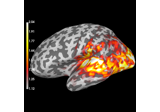Compute source power estimate by projecting the covariance with MNE

Compute source power estimate by projecting the covariance with MNE
pop(key, default=<unrepresentable>, /)#

If the key is not found, return the default if given; otherwise, raise a KeyError.

popitem(/)#

Remove and return a (key, value) pair as a 2-tuple.

Pairs are returned in LIFO (last-in, first-out) order. Raises KeyError if the dict is empty.

save(fname, *, overwrite=False, verbose=None)[source]#

Save covariance matrix in a FIF file.

Parameters:
fnamepath-like

Output filename.

overwritebool

If True (default False), overwrite the destination file if it exists.

New in v1.0.

verbose

Control verbosity of the logging output. If `None`, use the default verbosity level. See the logging documentation and `mne.verbose()` for details. Should only be passed as a keyword argument.

setdefault(key, default=None, /)#

Insert key with a value of default if key is not in the dictionary.

Return the value for key if key is in the dictionary, else default.

update([E, ]**F) None.  Update D from dict/iterable E and F.#

If E is present and has a .keys() method, then does: for k in E: D[k] = E[k] If E is present and lacks a .keys() method, then does: for k, v in E: D[k] = v In either case, this is followed by: for k in F: D[k] = F[k]

values() an object providing a view on D's values#

## Examples using `mne.Covariance`#Getting started with mne.Report

Getting started with mne.ReportWorking with CTF data: the Brainstorm auditory dataset

Working with CTF data: the Brainstorm auditory dataset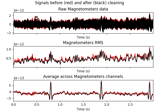Repairing artifacts with ICA

Repairing artifacts with ICA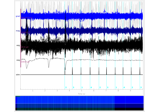Plotting whitened data

Plotting whitened dataComputing a covariance matrix

Computing a covariance matrix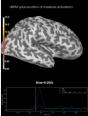Source localization with MNE, dSPM, sLORETA, and eLORETA

Source localization with MNE, dSPM, sLORETA, and eLORETA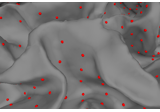The role of dipole orientations in distributed source localization

The role of dipole orientations in distributed source localization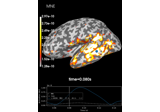Computing various MNE solutions

Computing various MNE solutionsSource reconstruction using an LCMV beamformer

Source reconstruction using an LCMV beamformer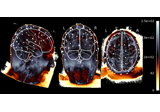EEG source localization given electrode locations on an MRI

EEG source localization given electrode locations on an MRI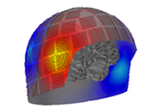Brainstorm Elekta phantom dataset tutorial

Brainstorm Elekta phantom dataset tutorial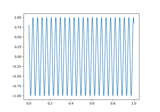Brainstorm CTF phantom dataset tutorial

Brainstorm CTF phantom dataset tutorial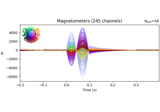4D Neuroimaging/BTi phantom dataset tutorial

4D Neuroimaging/BTi phantom dataset tutorial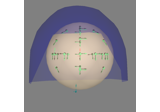KIT phantom dataset tutorial

KIT phantom dataset tutorial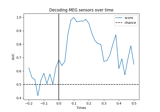Decoding (MVPA)

Decoding (MVPA)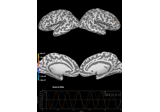Corrupt known signal with point spread

Corrupt known signal with point spread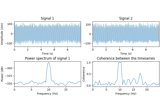DICS for power mapping

DICS for power mapping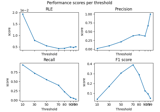Compare simulated and estimated source activity

Compare simulated and estimated source activity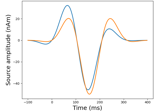Generate simulated evoked data

Generate simulated evoked data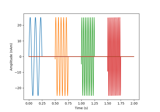Generate simulated raw data

Generate simulated raw data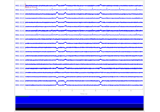Simulate raw data using subject anatomy

Simulate raw data using subject anatomy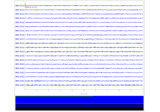Generate simulated source data

Generate simulated source data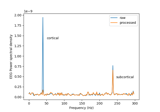Cortical Signal Suppression (CSS) for removal of cortical signals

Cortical Signal Suppression (CSS) for removal of cortical signals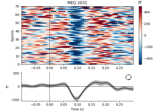XDAWN Denoising

XDAWN Denoising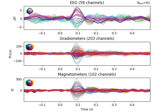Whitening evoked data with a noise covariance

Whitening evoked data with a noise covariance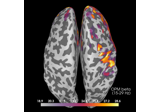Compute source power spectral density (PSD) of VectorView and OPM data

Compute source power spectral density (PSD) of VectorView and OPM data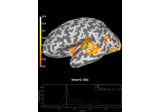Decoding source space data

Decoding source space data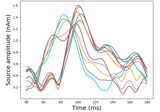Source localization with a custom inverse solver

Source localization with a custom inverse solverCompute evoked ERS source power using DICS, LCMV beamformer, and dSPM

Compute evoked ERS source power using DICS, LCMV beamformer, and dSPM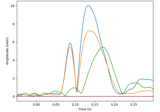Compute a sparse inverse solution using the Gamma-MAP empirical Bayesian method

Compute a sparse inverse solution using the Gamma-MAP empirical Bayesian method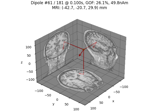Compute sparse inverse solution with mixed norm: MxNE and irMxNE

Compute sparse inverse solution with mixed norm: MxNE and irMxNE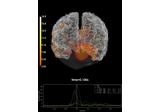Compute MNE inverse solution on evoked data with a mixed source space

Compute MNE inverse solution on evoked data with a mixed source spaceCompute source power estimate by projecting the covariance with MNE

Compute source power estimate by projecting the covariance with MNEComputing source timecourses with an XFit-like multi-dipole model

Computing source timecourses with an XFit-like multi-dipole model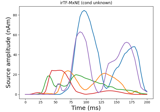Compute iterative reweighted TF-MxNE with multiscale time-frequency dictionary

Compute iterative reweighted TF-MxNE with multiscale time-frequency dictionary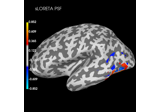Plot point-spread functions (PSFs) and cross-talk functions (CTFs)

Plot point-spread functions (PSFs) and cross-talk functions (CTFs)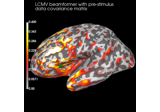Compute cross-talk functions for LCMV beamformers

Compute cross-talk functions for LCMV beamformers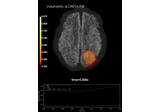Plot point-spread functions (PSFs) for a volume

Plot point-spread functions (PSFs) for a volume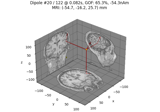Compute Rap-Music on evoked data

Compute Rap-Music on evoked data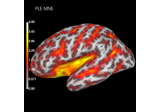Compute spatial resolution metrics in source space

Compute spatial resolution metrics in source space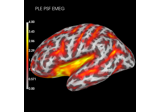Compute spatial resolution metrics to compare MEG with EEG+MEG

Compute spatial resolution metrics to compare MEG with EEG+MEG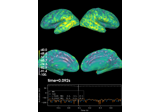Computing source space SNR

Computing source space SNR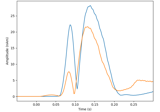Compute MxNE with time-frequency sparse prior

Compute MxNE with time-frequency sparse priorCompute Trap-Music on evoked data

Compute Trap-Music on evoked data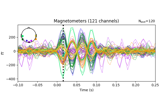Kernel OPM phantom data

Kernel OPM phantom data